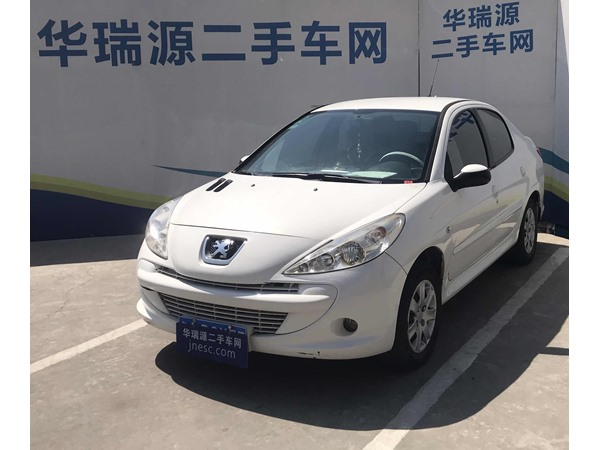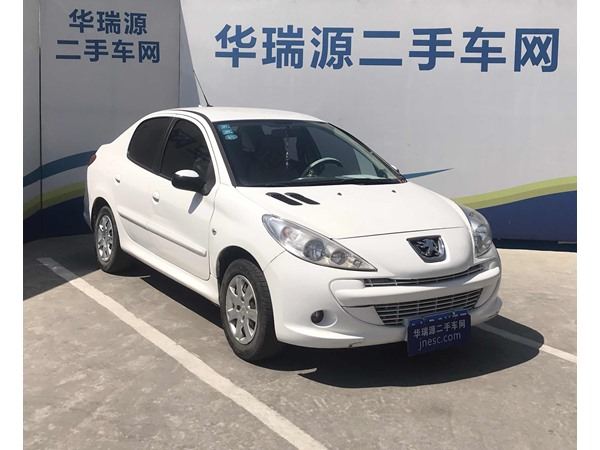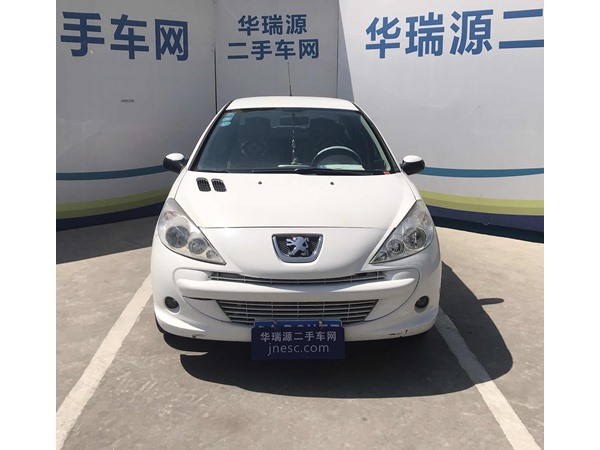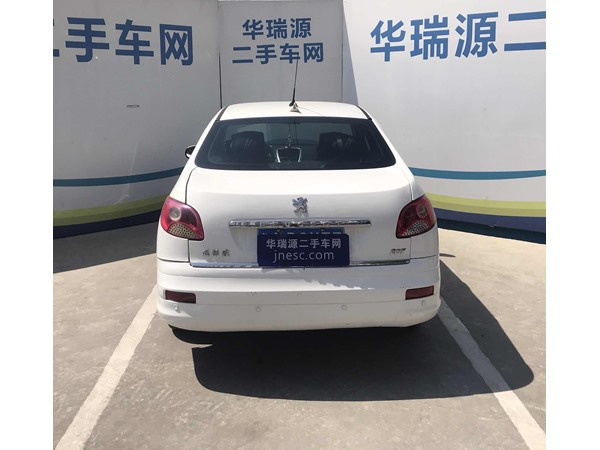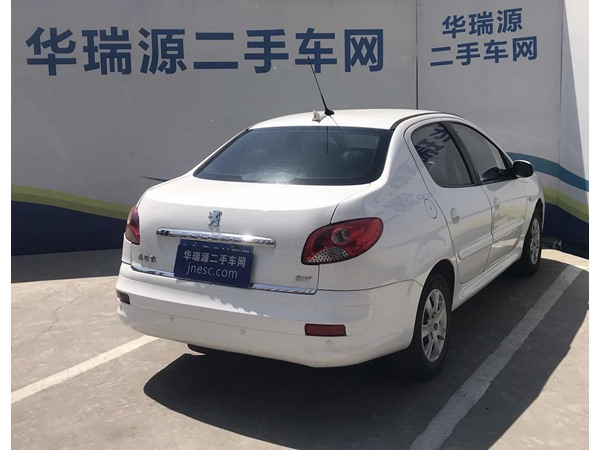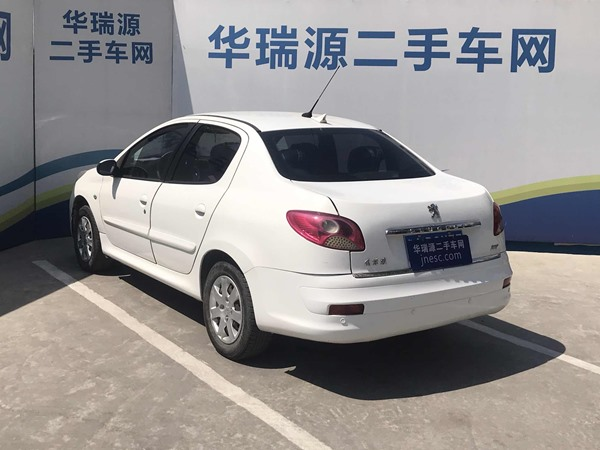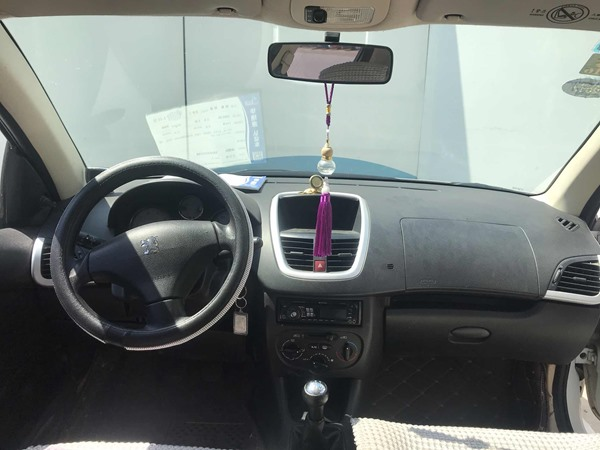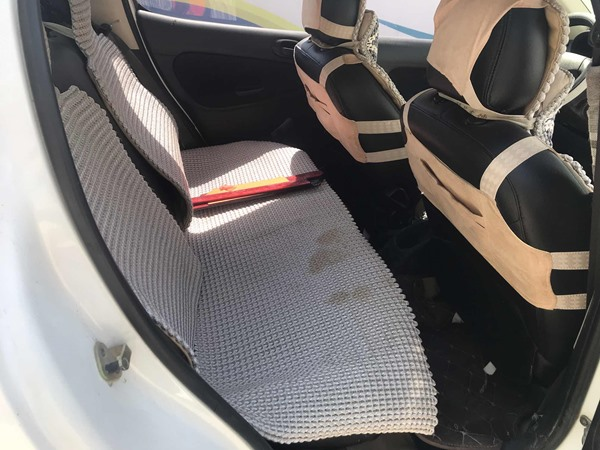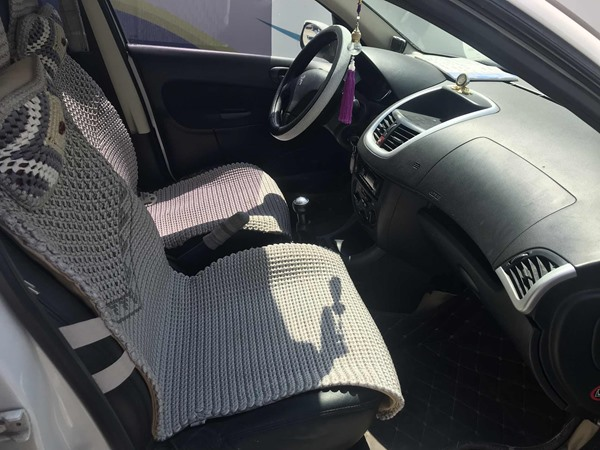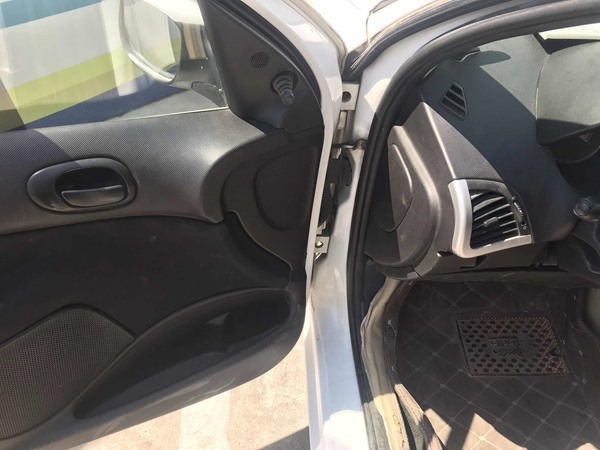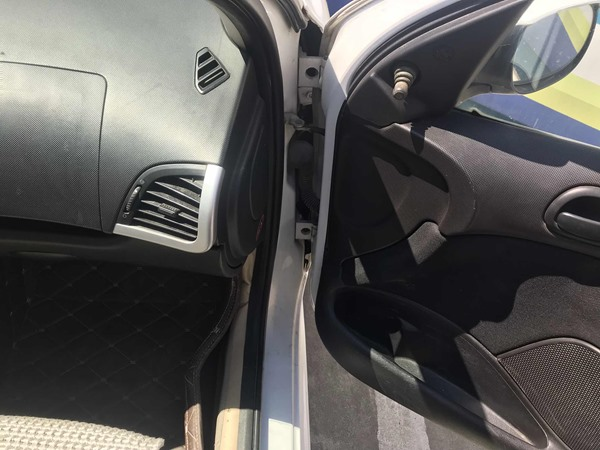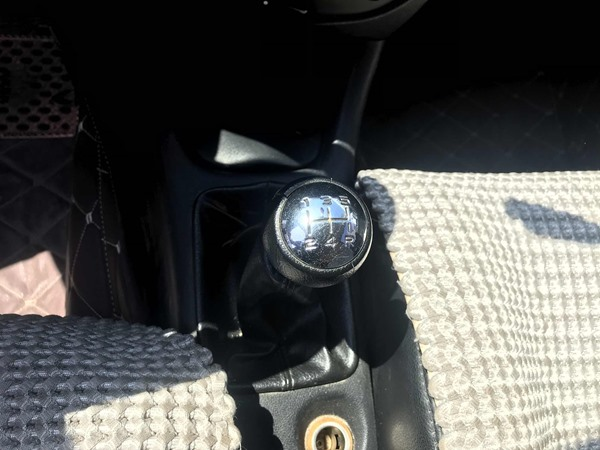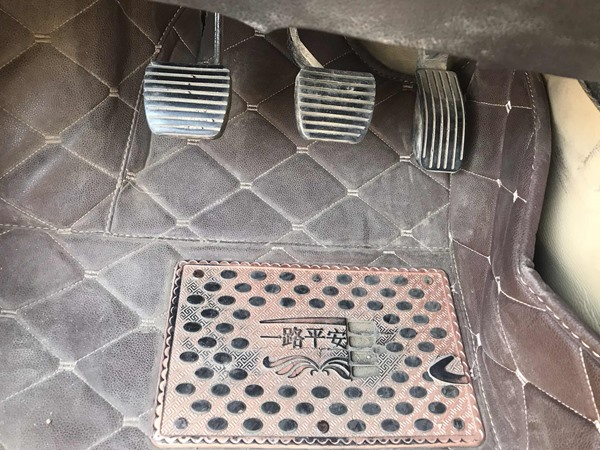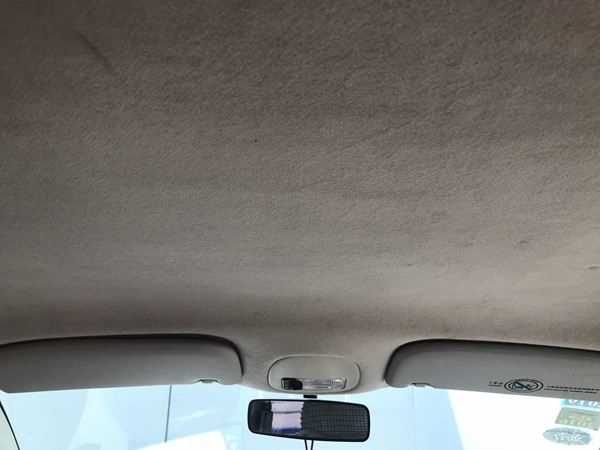车辆详情

标致 标致207 2009款 三厢 1.4L 手动驭乐版

1.15万   (包过户)   新车价： 7.48万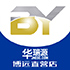• 2009-06
上牌时间
• 8.3万公里
表显里程
• 1.40L
排量
• 手动
变速箱
• 4
过户次数
• 济南市
所在地
• 国Ⅱ
环保标准
• 2019-12
保险到期张华卫*以上为初次检测时的车辆状况，交易以复检结果为准。

• 前机盖: -做漆

• 左前翼子板: -做漆

• 右前翼子板: -做漆

• 车顶:

• 左侧前门: -做漆

• 右侧前门: -做漆

• 左侧后门: -做漆

• 右侧后门: -做漆

• 后机盖: -做漆

• 左后翼子板: -做漆

• 右后翼子板: -做漆

• 左A柱:

• 右A柱:

• 左B柱:

• 右B柱:

• 左C柱: -做漆

• 右C柱: -做漆

• 前杠: -做漆

• 后杠: -做漆

• 左侧下边梁: -做漆

• 右侧下边梁: -做漆

• 左后视镜: -做漆

• 右后视镜: -做漆

• 左侧上边梁:

• 右侧上边梁:

• 车顶:

• 座椅:

• 地胶:

• 仪表盘:

• 方向盘:

• 挂挡杆:

• 扶手:

• 安全带:

• 车门:

• 备胎:

• 工具包:

• 千斤顶:

• 三脚架（警示牌）:

• 车辆钥匙:

• 左前大灯框架:

• 右前大灯框架:

• 前防撞梁:

• 后防撞梁:

• 左前翼子板内衬:

• 右前翼子板内衬:

• 左侧底边梁(金属):

• 右侧底边梁(金属):

• 左侧低大边(非金属):

• 右侧低大边(非金属):

• 水箱框架:

• 后备箱导水槽:

• 后围板:

• 左A柱:

• 右A柱:

• 左B柱:

• 右B柱:

• 左C柱:

• 右C柱:

• 左前纵梁:

• 右前纵梁:

• 左后纵梁:

• 右后纵梁:

• 左前减震器悬挂部位:

• 右前减震器悬挂部位:

• 左后减震器悬挂部位:

• 右后减震器悬挂部位:

• 车身底板:

• 后备箱底板:

• 防火墙:

• 车身左右对称性:

• 发动机线束及橡胶制品:

• 后备箱边角及备胎槽:

• 座椅滑轨内:

• 后排座椅坐垫底部:

• 安全带底部:

• 全车地胶地毯:

• 音响喇叭底部:

• 烟灰缸底座:

• 发动机线束及橡胶制品:

• 车辆覆盖件及驾驶舱:

• 安全带系统:

• 安全气囊系统:

• 防抱死系统(ABS):

• 车身稳定系统(ESP):

• 轮胎胎压:

• 儿童座椅接口:

• 刹车片:

• 制动卡钳:

• 驻车系统:

• 制动液:

• 车灯系统:

• 仪表指示灯:

• 雨刷器:

• 空调系统:

• 多媒体系统:

• 电动车窗:

• 中控锁:

• 座椅电动功能:

• 前悬挂:

• 后悬挂:

• 助力转向系统:

• 变速箱转动轴:

• 发动机:

• 排气系统检查:

• 左侧车身底边:

• 右侧车身底边:

• 车身底板:

• 发动机:

• 变速箱:

• 传动系统:

• 悬挂系统:

• 路试: